Finding rate of change

Chapter 6 Class 12 Application of Derivatives
Concept wise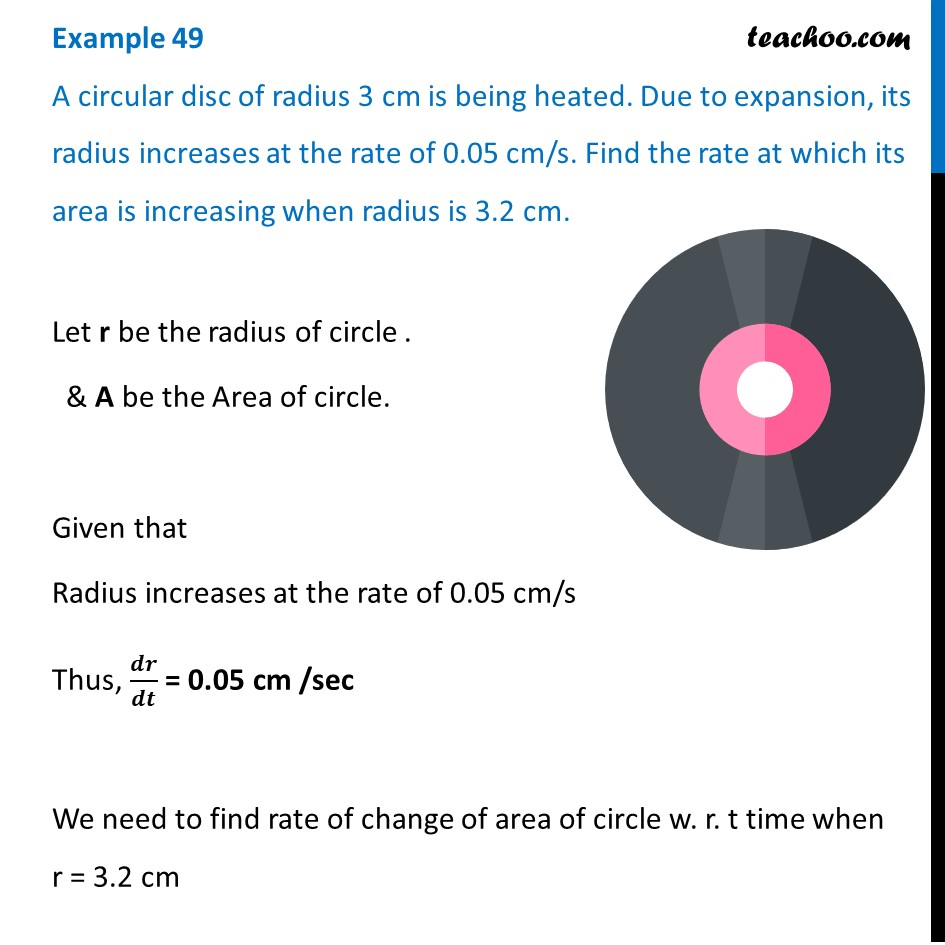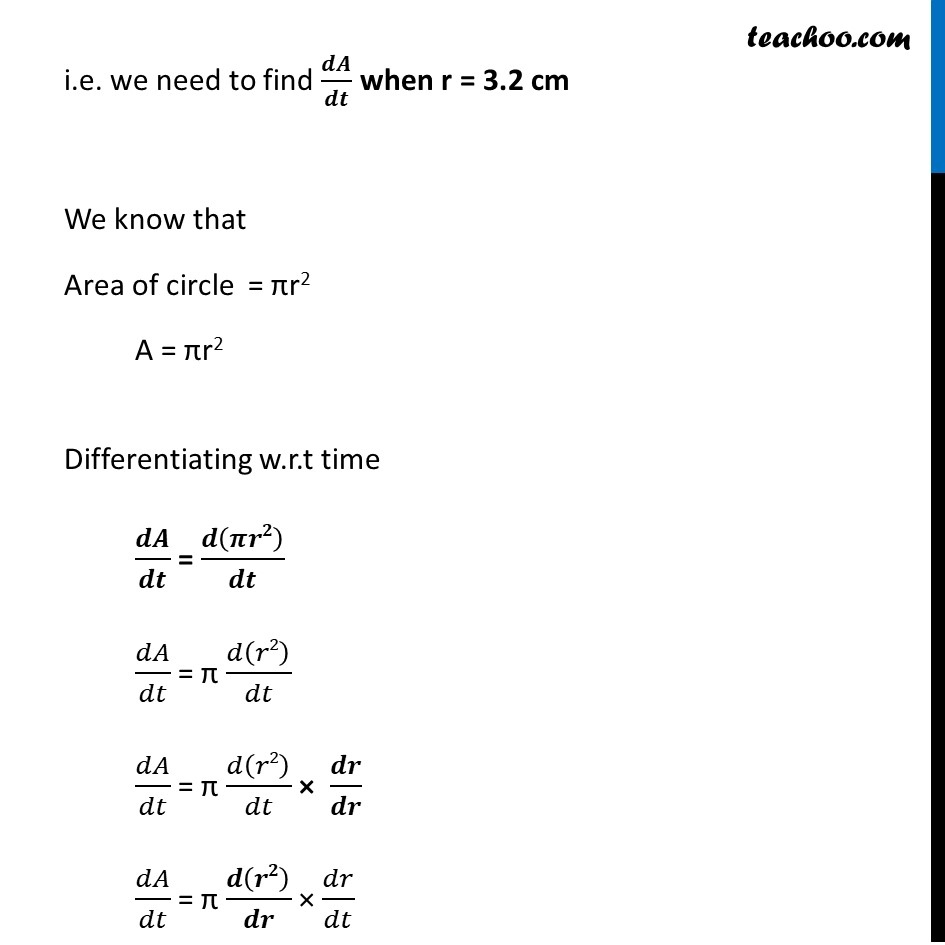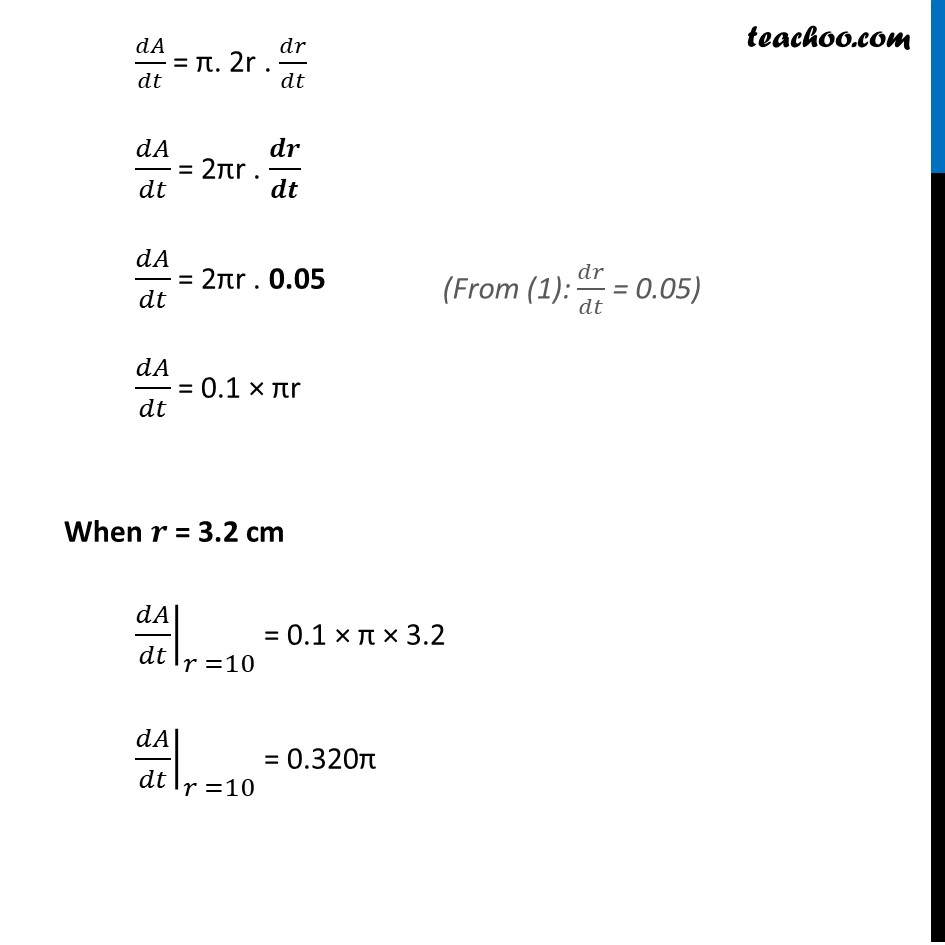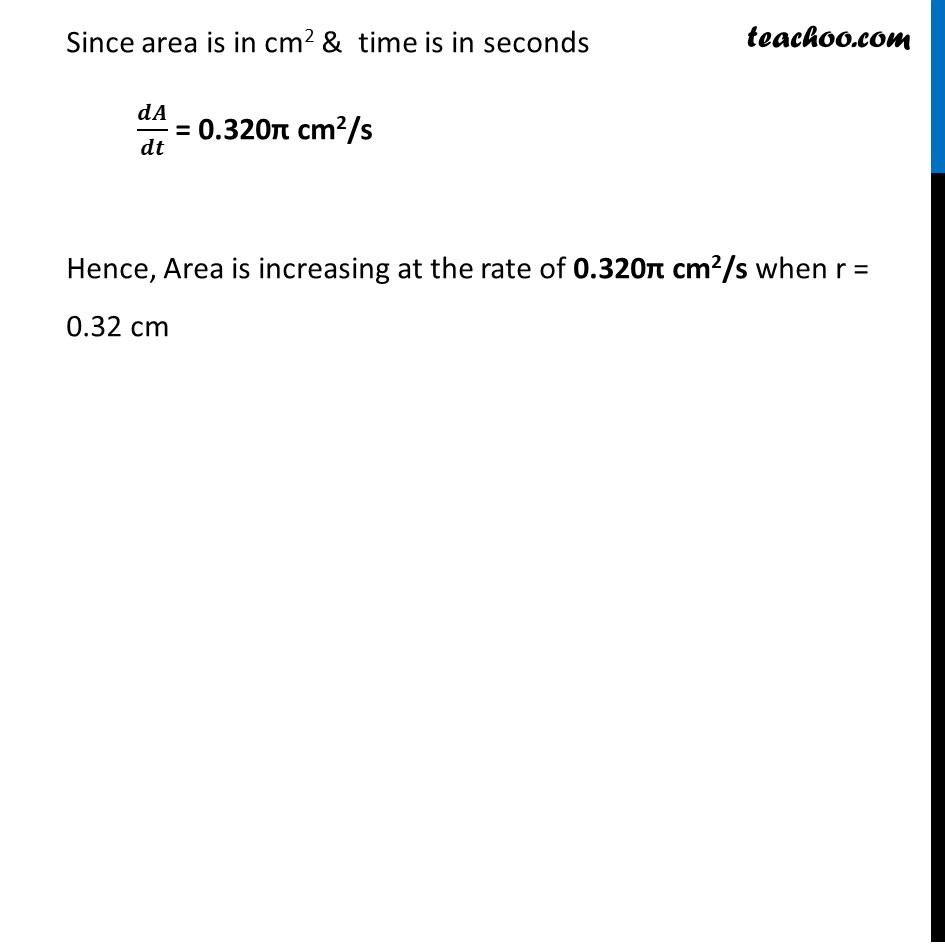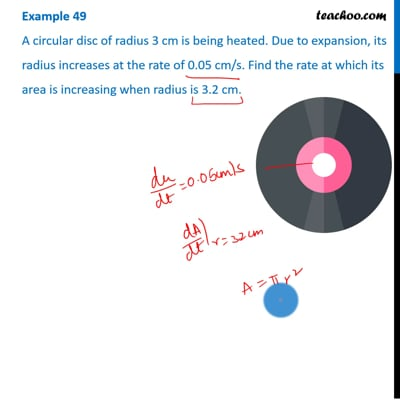This video is only available for Teachoo black users

Learn in your speed, with individual attention - Teachoo Maths 1-on-1 Class

### Transcript

Checking sign for numerator cos 𝑥 – sin 𝑥 > 0 cos 𝑥 > sin 𝑥 1 > sin⁡𝑥/cos⁡𝑥 1 > tan 𝑥 𝐭𝐚𝐧⁡𝒙<𝟏 This is possible only if 𝟎<𝒙<𝝅/𝟒 Thus, f’(𝒙) = ((+))/((+) ) > 0 in x ∈ (0 , 𝜋/4) Hence, f is strictly increasing function in (𝟎 , 𝝅/𝟒) Let r be the radius of circle . & A be the Area of circle. Given that Radius increases at the rate of 0.05 cm/s Thus, 𝒅𝒓/𝒅𝒕 = 0.05 cm /sec We need to find rate of change of area of circle w. r. t time when r = 3.2 cm i.e. we need to find 𝒅𝑨/𝒅𝒕 when r = 3.2 cm We know that Area of circle = πr2 A = πr2 Differentiating w.r.t time 𝒅𝑨/𝒅𝒕 = 𝒅(𝝅𝒓𝟐)/𝒅𝒕 𝑑𝐴/𝑑𝑡 = π 𝑑(𝑟2)/𝑑𝑡 𝑑𝐴/𝑑𝑡 = π 𝑑(𝑟2)/𝑑𝑡 × 𝒅𝒓/𝒅𝒓 𝑑𝐴/𝑑𝑡 = π 𝒅(𝒓𝟐)/𝒅𝒓 × 𝑑𝑟/𝑑𝑡 𝑑𝐴/𝑑𝑡 = π. 2r . 𝑑𝑟/𝑑𝑡 𝑑𝐴/𝑑𝑡 = 2πr . 𝒅𝒓/𝒅𝒕 𝑑𝐴/𝑑𝑡 = 2πr . 0.05 𝑑𝐴/𝑑𝑡 = 0.1 × πr When 𝒓 = 3.2 cm ├ 𝑑𝐴/𝑑𝑡┤|_(𝑟 =10) = 0.1 × π × 3.2 ├ 𝑑𝐴/𝑑𝑡┤|_(𝑟 =10) = 0.320π Since area is in cm2 & time is in seconds 𝒅𝑨/𝒅𝒕 = 0.320π cm2/s Hence, Area is increasing at the rate of 0.320π cm2/s when r = 0.32 cm